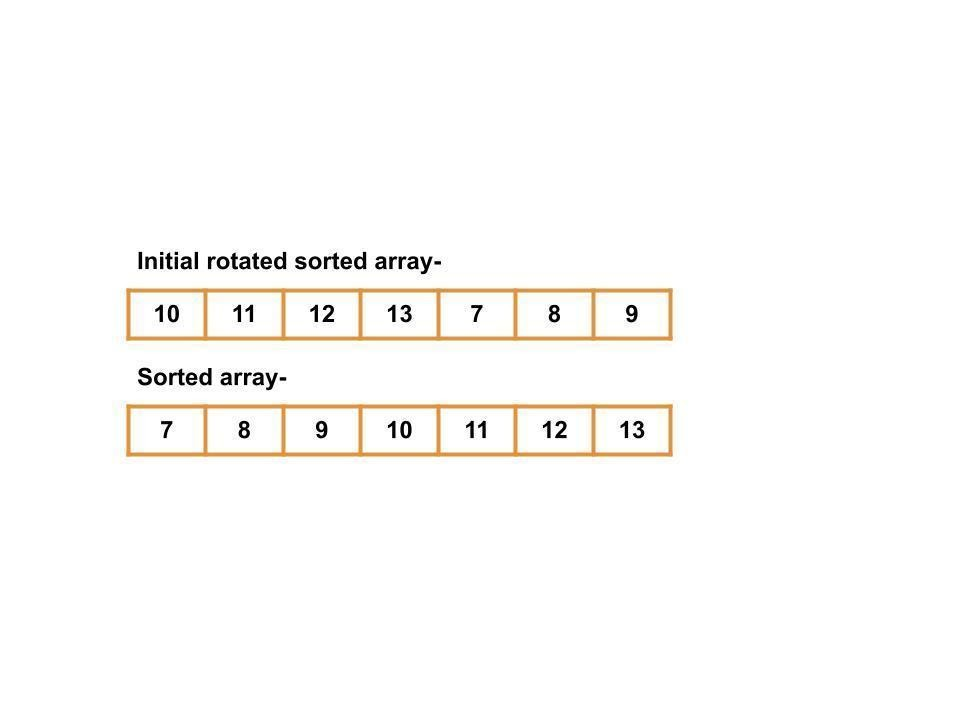# Sort a Rotated Sorted Array

## Introduction

Suppose we have a rotated sorted array of distinct elements, i.e., a sorted array rotated around some pivot. Now we are asked to restore the sorted array. Let's look at an example to understand this problem.In the above example, the initial array is a rotated sorted array, i.e., it is a sorted array rotated by three positions towards the left. Now, our task is to find the initially sorted array.

Though there could be numerous methods to solve this problem, we will focus on the two most commonly used ones. The first is a brute approach based on finding the pivot using linear search, splitting the array around it, reversing the subarrays, then reversing the whole array to get the sorted array.

The second method is the optimization of the first method. In this method, we use binary search to find the array's pivot element. Then split and reverse the arrays, as we did in the first method. Let's understand these methods one by one-

## The basic solution

The basic solution of this problem starts with finding the pivot of the array. To do so, we use Linear search in which we traverse the array from left to right and check each element if it is smaller than its predecessor. After finding the pivot, we split the array into two subarrays. Left subarray from the first element to the predecessor of the pivot element. Right subarray from the pivot element to the last element of the array. Then we first reverse these subarrays, after which we reverse the whole array to get the initially sorted array.

### Algorithm

1. Take the array and key from user input.
2. Traverse the array to find the pivot element
3. call the reverse function in the following order-
• From the 0th index to (pivot-1) index
• From the (pivot) index to the last index.
• From the 0th index to the last index.
1. Print the array.

### Implementation of the basic approach

Input-

Output-

The time complexity of this algorithm is O(N), as we are using binary search.

The space complexity of this algorithm is O(1), as no extra space is required.

## The optimized approach

The second method is an optimized version of the basic approach. In this method, we use binary search instead of using linear search to find the pivot element. It takes O(logN) time for searching than linear search's O(N)  time. The rest of the steps are similar to the basic approach.

### Algorithm

1. Take the array and key from user input.
2. Use the binary search in the array to find the pivot element, which will be used as a pivot to split the array.
3. call the reverse function in the following order-
• for the 0th index to (pivot-1) and the (pivot) to the last index.
• For the whole array.
1. Print the array.

### Implementation of the optimized approach

Input-

Output-

The time complexity of this algorithm is O(N), as the reverse function in c++, stl has a time complexity of O(N)

The space complexity of this algorithm is O(1), as no extra space is required.

1. How do you check if an array is sorted and rotated?
To check if an array is sorted and rotated, we traverse the array from left to right. If the initial array were sorted in increasing order, only one element would be smaller than its previous one. The first element is always greater than the last element in a rotated sorted array.

2. What is a circularly sorted array?
A circularly sorted array means that the arrays are initially sorted, but few elements rotate it. And during rotation, the element at the first moves to the last position in clockwise rotation, or the last element moves to the first element in an anti-clockwise rotation.

3. What is a pivot in a rotated array, and how do you find it?
The pivot element is the only element in the array which is smaller than its previous element. We can find it by searching the array for an element that is smaller than its previous element. We can use multiple methods like linear search or binary search.

4. How can you tell if an array is sorted?
If every element in an array is either greater than or equal to its predecessor, the array is sorted.

5. Where is the pivot in a rotated sorted array?
Pivot is at such a position in the rotated sorted array that if we divide the array into two subarrays, one from the first element to the predecessor of the pivot, the other from the pivot element to the last element, both the array will be sorted. Also, in a rotated sorted array, the first element can never be the pivot as the array will already be in a sorted state if the first element is the pivot.

## Key takeaways

In this blog, we learned about sorting rotated arrays of distinct elements. To achieve this task, we used two methods-

• We started with a simple approach. We first find the pivot element in the array then divide the array into subarrays around that element. After that, we reverse each of the subarrays, then reverse the whole array.
• The second approach was an optimized version of the first approach. However, the idea of splitting the array into two subarrays and reversing the array is the same as the basic approach. But instead of using linear search to find the pivot element, we use binary search. Then we follow a similar procedure as the first method to sort the array.

Visit here to learn more about arrays. And practice similar problems on CodeStudio. If you liked this blog, share it with your friends.